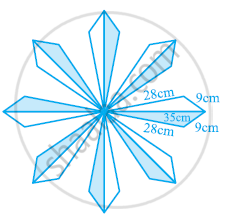# A floral design on a floor is made up of 16 tiles which are triangular, the sides of the triangle being 9 cm, 28 cm and 35 cm (see the given figure). Find the cost of polishing the tiles at the rate of 50p per cm^2. - Mathematics

A floral design on a floor is made up of 16 tiles which are triangular, the sides of the triangle being 9 cm, 28 cm and 35 cm (see the given figure). Find the cost of polishing the tiles at the rate of 50p per cm2.#### Solution

It can be observed that

Semi-perimeter of each triangular-shaped tile,

s=(35+28+9)/2=36cm

By Heron’s formula,

"Area of triangle "=sqrt(s(s-a)(s-b)(s-c))

"Area of each tile "=[sqrt(36(36-35)(36-28)(36-9))]cm^2

=[sqrt(36xx1xx8xx27)]cm^2

=36sqrt6 cm^2

= (36 x 2.45) cm^2

= 88.2 cm2

Area of 16 tiles = (16 × 88.2) cm2= 1411.2 cm2

Cost of polishing per cm2 area = 50 p

Cost of polishing 1411.2 cm2 area = Rs (1411.2 × 0.50) = Rs 705.60

Therefore, it will cost Rs 705.60 while polishing all the tiles.

Concept: Application of Heron’s Formula in Finding Areas of Quadrilaterals
Is there an error in this question or solution?
Chapter 12: Heron's Formula - Exercise 12.2 [Page 207]

#### APPEARS IN

NCERT Class 9 Maths
Chapter 12 Heron's Formula
Exercise 12.2 | Q 8 | Page 207

Share